# Introduction to careless¶

## Authors: Francisco Wilhelm & Richard D. Yentes¶

Date: June 10, 2018

The careless package provides functions that help you screen survey data for types of responses called "careless" or "insufficient effort". These response types will lower the quality of the data, that, if unchecked, will distort data analysis and, in turn, lead to false inferences drawn from the data. This documents provides a short introduction to the basic functions of the package and teaches you how to screen your data for careless responses.

## Installation¶

Install from github

devtools::install_github('ryentes/careless')

## Sample Dataset: Simulation study¶

The package comes with two simulated datasets, careless::careless_dataset and careless::careless_dataset2. We will use careless_dataset2 in this introduction. The documentation of this dataset can be accessed via ?careless_dataset2.

In order to simulate these data, we began with a public dataset of responses to the IPIP HEXACO measure, which was downloaded from ‘http://personalitytesting.info/_rawdata/’. The dataset consists of 240 items spread across six factors, each with four facets. A subset of 10 facets were chosen from across the factors to serve as a 100-item measure. Correlations between each of the selected facets were computed. IRT item parameters for the graded response model were estimated for each facet using the MIRT package in R (Chalmers, 2012). Using the parameters obtained from estimation, a dataset of careful respondents was simulated. A number of careless respondents were then simulated and assigned randomly to replace careful respondents. More information about the data simulation procedures is available upon request.

## The classic: Longstring Index¶

Perhaps the most straightforward index to detect careless responses is the Longstring index, available via longstring(). It is defined as the longest consecutive string of identical responses given by a person. For example, imagine a person giving the following answers to a Likert scale with 7 items:

In :
x <- c(4,4,4,3,3,3,3,3,4,4)
print(x)

  4 4 4 3 3 3 3 3 4 4


The longest string of identical responses would be 5, since the person gives the same response ("3") five times in a row. The logic behind the longstring index is simple: If a person gives the same response consecutively over a long stretch of items, this can be taken as an indication of careless responding. In the literature, such a response type is often called "straightlining".

Now, let us apply longstring() to our sample dataset.

In :
careless_long <- longstring(careless_dataset2)
boxplot(careless_long, main = "Boxplot of Longstring index")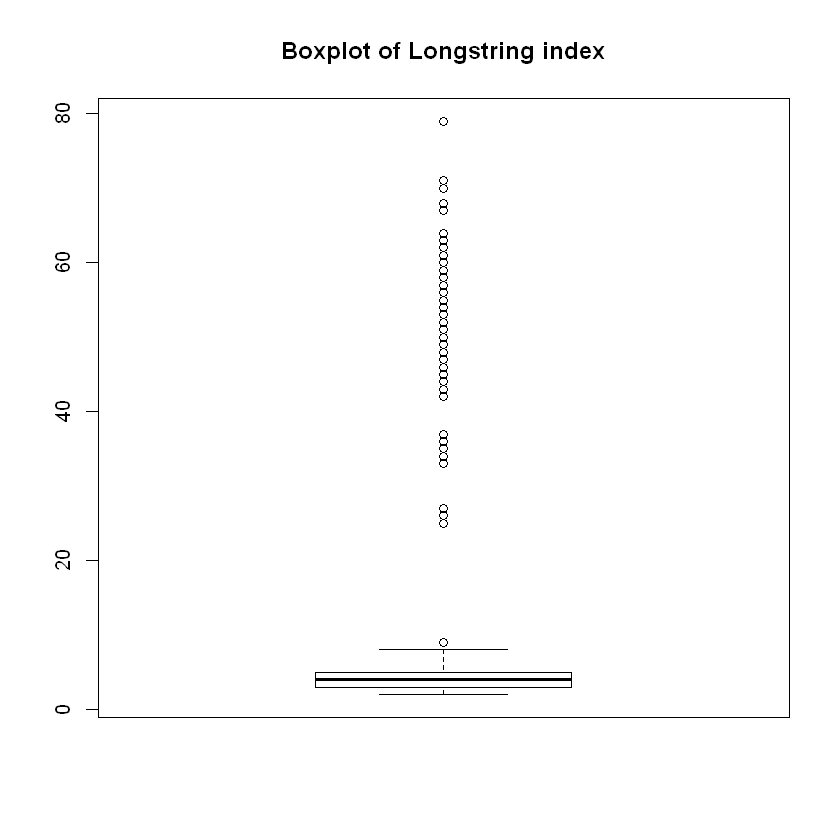The boxplot shows that some observations clearly have very high Longstring values, indicating that they might be careless responders. The problem of appropriate cut-off values for careless responding is a tricky one, and no general rule of thumb can be given. We will return to it later.

The longstring() function also comes with an additional variant that calculates the average length of consecutive identical responses - let us call this index Averagestring. Recall the previous example:

In :
print(x)

  4 4 4 3 3 3 3 3 4 4


The Longstring for this observation would, as we have seen, be 5, and the Averagestring would be $\frac{3+5+2}{3} = 3.33$ . The Averagestring Index helps spotting persons who exercise a bit more effort than the classical "straightliner" - alternating their responses more often.

In :
careless_long <- longstring(careless_dataset2, avg = T)
boxplot(careless_long$avg, main = "Boxplot of Averagestring index")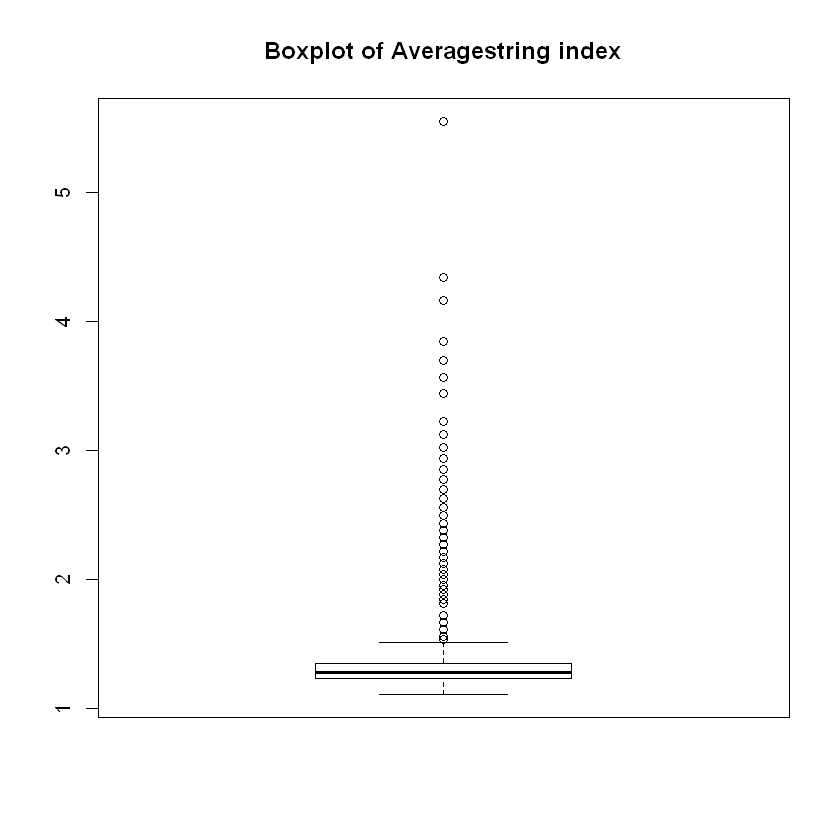The boxplot shows some persons with high values Averagestring. The most extreme observation has an average of above 5, and calls for further inspection. ## Intra-individual Response Variability¶ The intra-individual response variability (IRV) is similiar in spirit to the Longstring index. It is defined as the "standard deviation of responses across a set of consecutive item responses for an individual" (Dunn et al. 2018). Consider this example of an individual with the following set of item responses: In : x <- c(4,5,4,5,4,5,4,5,4,5) print(x)    4 5 4 5 4 5 4 5 4 5  The individual alternates between responding with option 4 and option 5. Neither the Longstring nor the Averagestring would detect such a response pattern, with a value of "1" for both. The IRV might, however, detect this response pattern because the value is rather low: In : sd(x)  0.52704627669473 Hence, the purpose of the IRV is to detect these kinds of invariability in responding that are hard to detect using Longstring. The IRV can be calculated by calling irv(). Dunn et al. (2018) propose that the IRV also be calculated for subsets of the questionnaire in order to detect careless responding that occurs only during a subsection of the questionnaire (e.g., at the end, when ego depletion has occured). Rather than manually splitting the questionnaire and feeding it to the function piecewise, you can do this by calling irv() with the arguments split = TRUE and num.split = n, where n is the number of subsets you want to split the dataset into. The function will try to split the dataset into subsets of equal lengths. In : careless_irv <- irv(careless_dataset2, split = TRUE, num.split = 4) head(careless_irv)  irvTotalirv1irv2irv3irv4 2.1278342.6057632.0000001.7058722.160247 1.7507292.2271061.8101571.2583061.107550 1.7222251.9295941.4854851.7795131.719496 1.9427152.0840671.8947301.7349351.908752 1.7872731.5989582.1855591.7320511.630951 1.9049112.0355181.7243361.6832512.051828 As shown, the function then returns both the IRV for the whole dataset and for each subset. ## Psychometric Synonyms and Antonyms¶ The Psychometric Synonyms index takes variable pairs (these should be items) that are highly correlated (e.g.,$r > .60$), and in that sense psychometrically synonymous, and calculates a within-person correlation over these item pairs. The rationale behind this index is that pairs of items that elicit similar responses across the population should also do so for each individual. To create the index, the user has to set a sound critical value or cut-off value, above which a correlation between item pairs constitutes a pair of psychometric synonyms. The function psychsyn_critval() assists here: It returns a list of all possible correlations between variables, ordered by magnitude. In : psychsyn_cor <- psychsyn_critval(careless_dataset2) head(psychsyn_cor)  Var1Var2Freq 6668APati8 APati7 0.7893989 6567APati7 APati6 0.7707801 6568APati8 APati6 0.7365130 6669APati9 APati7 0.7167873 7073CPerf3 CPerf1 0.7106777 6769APati9 APati8 0.7049997 There is no clear answer on how to set this critical value. A rule of thumb often proposed in the literature is$r > .60$. In : sum(psychsyn_cor$Freq > .60, na.rm = TRUE)

31

In our sample dataset, there are 31 item pairs given this rule of thumb, a good size for our next step. If the number of item pairs is too low, the resulting index will have a low validity. In such cases you might want to consider a different critical value, but this again can be detrimental to validity.

Next, we call the function psychsyn() to calculate the psychometric synonyms index.

In :
example_psychsyn <- psychsyn(careless_dataset2, critval = .60)
hist(example_psychsyn, main = "Histogram of psychometrical synonyms index")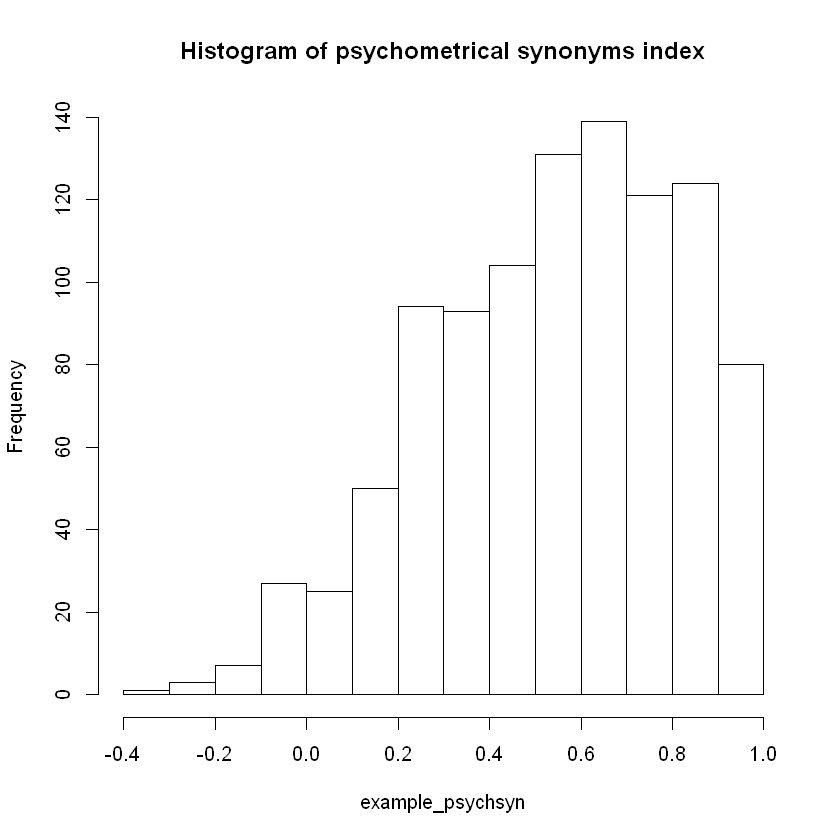Note that the histogram shows a distribution centered around $r_{within} = 0.6$. Observations whose correlations are close to zero or even negative should be inspected more closely.

Similarly, one can compute the Psychometric Antonyms index via psychant() or by calling psychsyn() with the argument anto = TRUE. Psychometric antonyms are item pairs which are correlated highly negatively. Otherwise, this index functions in the same way as the Psychometric Synonyms index. Finding psychometric antonyms is usually more tricky than finding psychometric synonyms, and often relies on reverse-worded items that correlate negatively with items from their scale.

In :
psychant_cor <- psychsyn_critval(careless_dataset2, anto = TRUE)

Var1Var2Freq
1018HFair8 HFair1 -0.6290865
2126EAnxi6 EAnxi2 -0.6242194
2226EAnxi6 EAnxi3 -0.5951980
1318HFair8 HFair4 -0.5882713
1017HFair7 HFair1 -0.5852945
1317HFair7 HFair4 -0.5683951

In our sample dataset, there are only two item pairs that exceed a threshold of $r < -.60$. In this case, we would either need to justify using a different critical value or abstain from using psychological antonyms. ​

## Even-Odd Consistency Index¶

The Even-Odd Consistency Index operates in the following manner:

1. divides unidimensional scales in two halves using an even-odd split
2. two scores, one for the even and one for the odd subscale, are then computed as the average response across subscale items
3. a within-person correlation is computed based on the two sets of subscale scores for each scale (think of the even scores as the variable $x$, the odd scores as the variable $y$ of a correlation $r(x,y)$)
4. the correlation is corrected for decreased length of the scale using the Spearman–Brown prophecy formula. ​ If persons consistenly rate the items of a unidimensional scale in a similar manner, we can expect their score on the even-odd consistency index to be high; if they are inconsistent, we can expect their score to be closer to zero. ​ This index is computed using the function evenodd(). In the function call, a vector of integers specifying the length of each scale in the dataset is supplied with the argument factors. As stated in ?careless_dataset2, our sample dataset includes 10 scales of 10 items each.
In :
careless_eo <- evenodd(careless_dataset2, factors = rep(10,10))
hist(careless_eo, main = "Histogram of even-odd consistency index")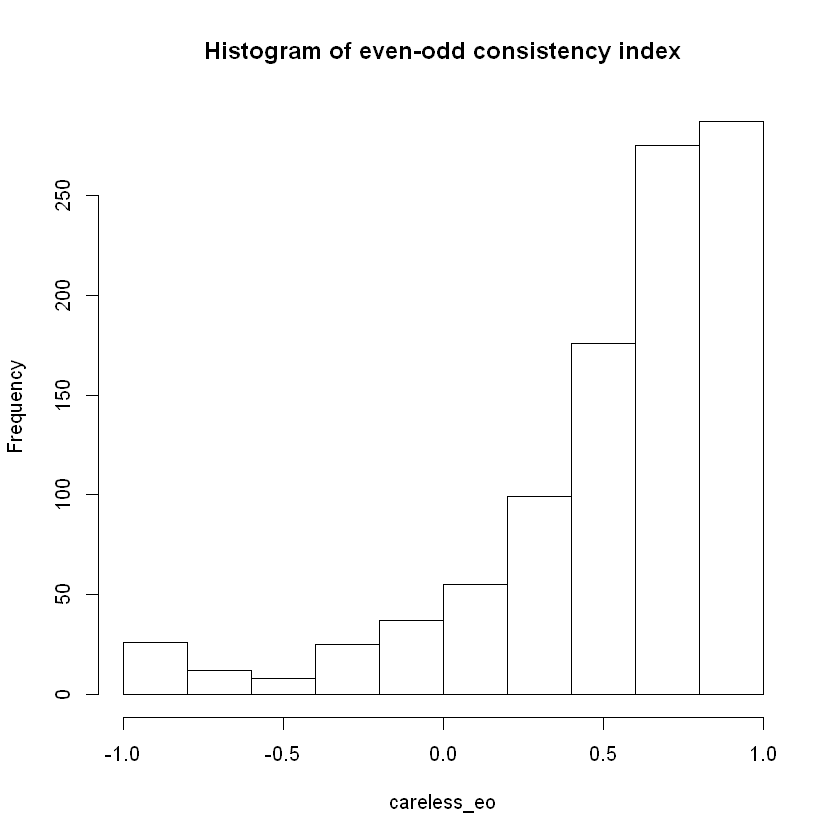Note that some scores are around $r_{within} = 0$ or even negative, although the scales are meant to be unidimensional. Some of these persons probably attribute a different meaning to the items of these scales, resulting in lower scores, but other persons might be careless responders.

## Mahalanobis Distance¶

Mahalanobis Distance or simply Mahalanobis $D^2$ is a method for multivariate outlier detection. Careless responding is just one reason why a person's set of answers to a survey may make her an outlier. Mahalanobis $D^2$ is thus not specifically adressed towards careless responding, but has been shown to be valuable for this problem. Summarized shortly, Mahalanobis $D^2$ measures the distance of a point (the set of responses from a person) to a distribution (usually the set of responses of all persons in a dataset). It is a multivariate generalization of the basic, univariate approach to outlier analysis where one looks at how many standard deviation a person is away from the mean on a single variable. If a person engages in careless responding, it is reasonable to expect that her responses will significantly deviate from the mean of the distribution of responses of others (given that most persons in a dataset are careful responders). Like in the univariate case, the closer to zero a person's value on Mahalanobis $D^2$ is, the closer a person is to the mean of the distribution. A high value on Mahalanobis $D^2$, on the other hand, indicates a large deviation from the mean that needs further attention. Mahalanobis $D^2$ can be calculated by calling mahad(). If no argument is passed, its output also includes a quantile-quantile (Q-Q) plot to help visually detect outliers.

In :
careless_mahad <- mahad_raw <- mahad(careless_dataset)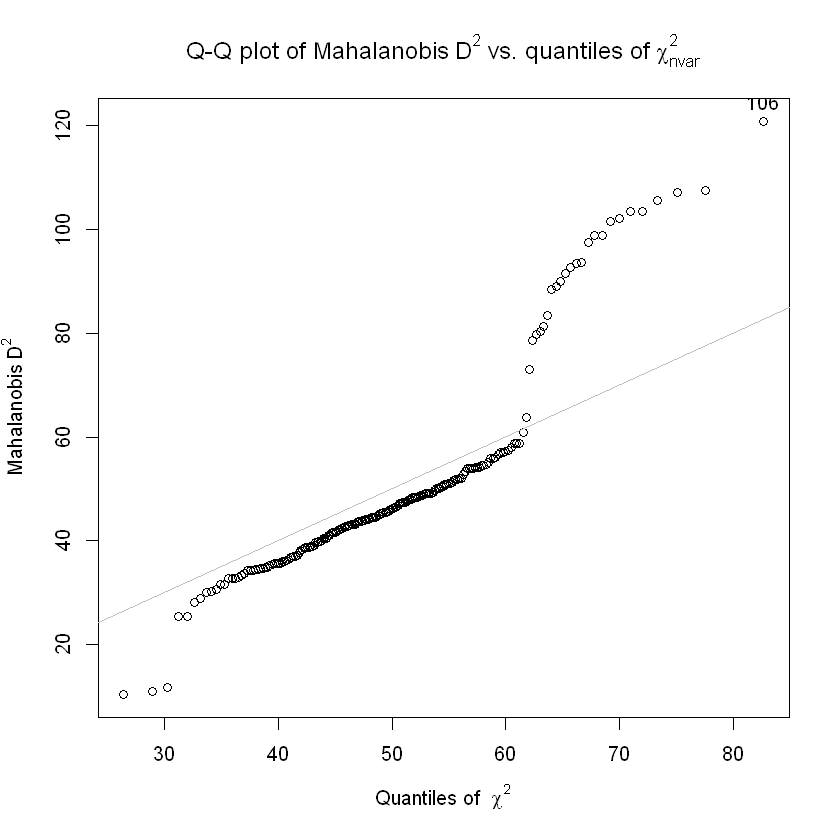The Q-Q plot shows the quantiles of the empirical distribution of Mahalanobis $D^2$ in the dataset vs. the quantiles of the theoretical distribution of $X^2_{nvar}$. Given no outliers, one would expect that the quantiles match each other such that points remain close to the gray line. The plot shows that starting at roughly the 60th quantile, the empirical values of Mahalanobis $D^2$ start to deviate strongly from the line. These values call for further inspection.